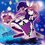Source: Grade 10 Selection Test for Gifted Math Students, Academic Year 2016 - 2017, High School for the Talented, HCMC, Vietnam.

Here I post the entire test. Among of them are some problems which I couldn't solve when doing the test (so hard!). Try to solve if you are interested.

1. Type-2 Symmetric System of Equations:

Given this system $\begin{cases} \$$x-2y)(x+my)=m^2-2m-3 \\(y-2x)(y+mx)=m^2-2m-3 \end{cases}$ ($x,y$ are variables, $m$ is a parameter). • Solve the system when $m=-3$. • Find $m$ so that the system has at least 1 solution $(x_0;y_0)$ satisfy $x_0>0$ and $y_0>0$. 2. Ugly Vieta's in Quadratic Equation: Find $a \geq 1$ so that the equation $ax^2+(1-2a)x+1-a=0$ has 2 different solutions $x_1,x_2$ satisfy $x_2^{2}-ax_1=a^2-a-1$. 3. Vieta Jumping, Where Are You? Let $x,y$ be positive integers satisfy $xy|x^2+y^2+10$. • Prove that $x,y$ are coprime odds. • Let $k=\dfrac{x^2+y^2+10}{xy}$. Prove that $4|k$ and $k \geq 12$. • Bonus (not in the test): How many different values of $k$ are there? 4. Inequality: Let $x \geq y \geq z$ be reals satisfy $x+y+z=0$ and $x^2+y^2+z^2=6$. • Find $S=(x-y)^2+(x-y)(y-z)+(y-z)^2$. • Find the maximum value of $P=|(x-y)(y-z)(z-x)|$. 5. Geometry - Welcome to Russia: Acute triangle $ABC$ with $\angle BAC > 45^\circ$. Draw squares $ABMN, ACPQ$ outside the triangle $ABC$. $AQ$ intersects $BM$ at $E$. $AN$ intersects $CP$ at $F$. • Prove that triangle $ABE$ and triangle $ACF$ are similar. Prove that $EFQN$ is concylic. • Let $I$ be midpoint of segment $EF$. Prove that $I$ is the circumcenter of triangle $ABC$. • $MN$ intersects $PQ$ at $D$. $(DMQ)$ and $(DNP)$ intersect at $K \neq D$. Tangents of $(ABC)$ at $B$ and $C$ intersect at $J$. Prove that $D, A, K, J$ are colinear. Clarification: $(\cdot)$ denotes the circumcircle of the figure. 6. Integral Sequence About Factorization: For each positive integer $x$ greater than $1$, let $f(x)$ be the second greatest factor of $x$. For example, $f(10)=5, f(45)=15, f(p)=1$ for any prime $p$. Consider the following sequence $\left\{ u_n \right\}: \begin{cases} \\u_0 \text{ is given} \\u_{n+1}=u_n-f(u_n) \end{cases}$. • Prove that for any given value of $u_0$, there exists an integer $k$ such that $u_k=1$. • (Is this too large?) Find $k$ if $u_0=2^{2016} \times 14^{2017}$.Note by Tran Quoc Dat 5 years, 1 month ago This discussion board is a place to discuss our Daily Challenges and the math and science related to those challenges. Explanations are more than just a solution — they should explain the steps and thinking strategies that you used to obtain the solution. Comments should further the discussion of math and science. When posting on Brilliant: • Use the emojis to react to an explanation, whether you're congratulating a job well done , or just really confused . • Ask specific questions about the challenge or the steps in somebody's explanation. Well-posed questions can add a lot to the discussion, but posting "I don't understand!" doesn't help anyone. • Try to contribute something new to the discussion, whether it is an extension, generalization or other idea related to the challenge. • Stay on topic — we're all here to learn more about math and science, not to hear about your favorite get-rich-quick scheme or current world events. MarkdownAppears as *italics* or _italics_ italics **bold** or __bold__ bold - bulleted- list • bulleted • list 1. numbered2. list 1. numbered 2. list Note: you must add a full line of space before and after lists for them to show up correctly paragraph 1paragraph 2 paragraph 1 paragraph 2 [example link](https://brilliant.org)example link > This is a quote This is a quote  # I indented these lines # 4 spaces, and now they show # up as a code block. print "hello world" # I indented these lines # 4 spaces, and now they show # up as a code block. print "hello world" MathAppears as Remember to wrap math in \( ... $$ or $ ... $ to ensure proper formatting.
2 \times 3 $2 \times 3$
2^{34} $2^{34}$
a_{i-1} $a_{i-1}$
\frac{2}{3} $\frac{2}{3}$
\sqrt{2} $\sqrt{2}$
\sum_{i=1}^3 $\sum_{i=1}^3$
\sin \theta $\sin \theta$
\boxed{123} $\boxed{123}$